# 如何使用 COMSOL Multiphysics® 分析真空系统中的稀薄气体

2021年 1月 12日

### 真空系统简介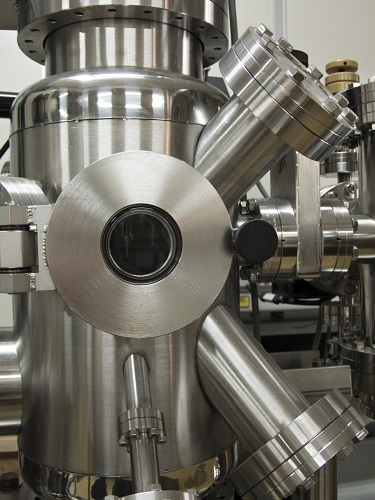• 旋片泵
• 定片泵
• 扩散泵
• 涡轮分子泵
• 低温泵
• 离子泵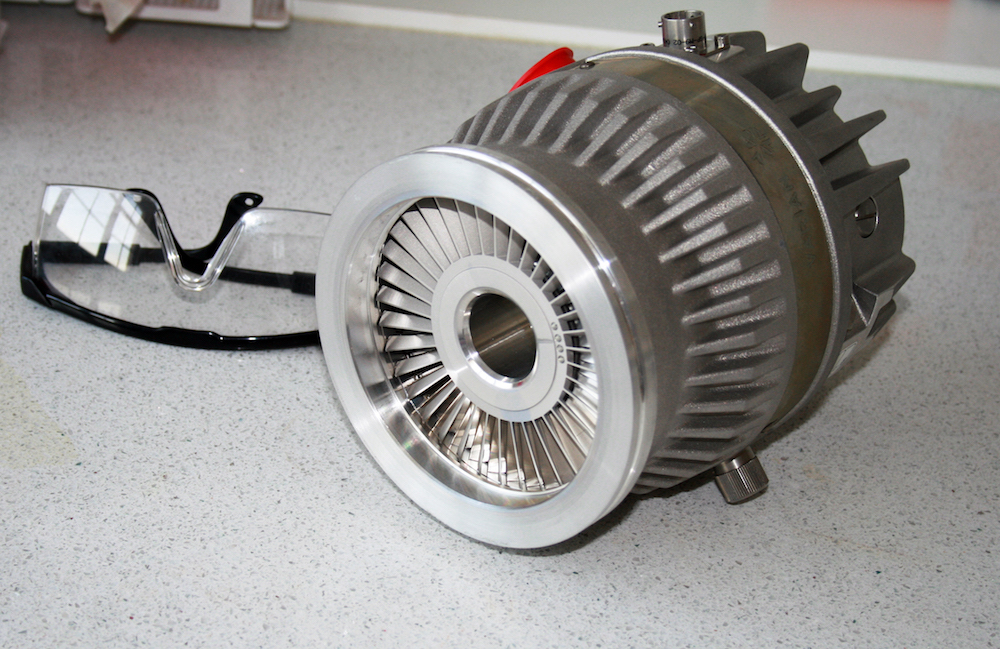### 真空系统中的稀薄气流### 涡轮分子泵详解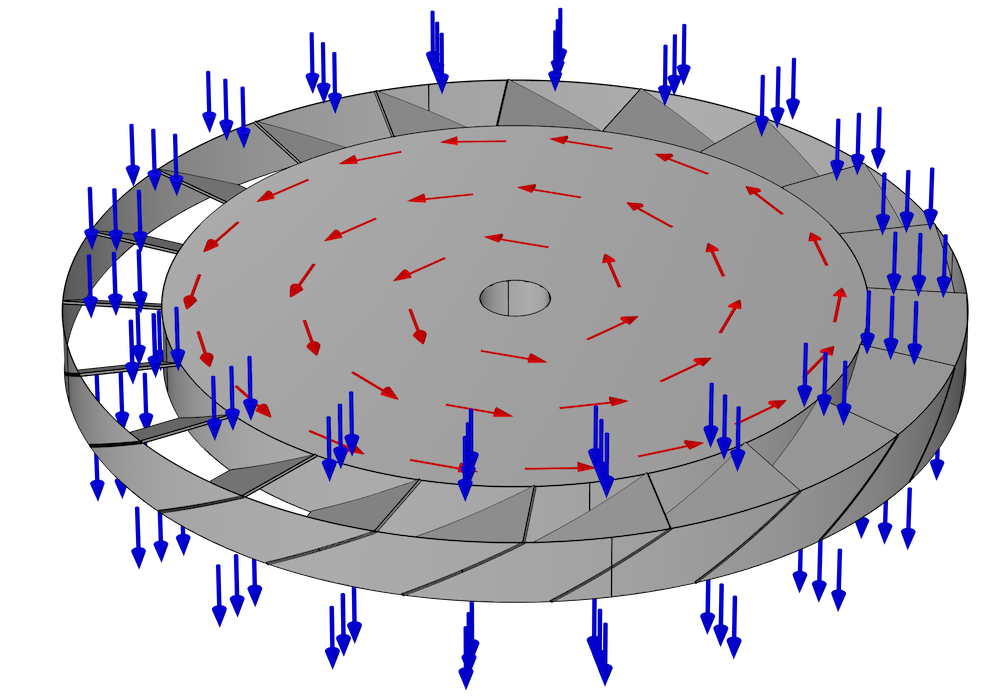### 选择数值方法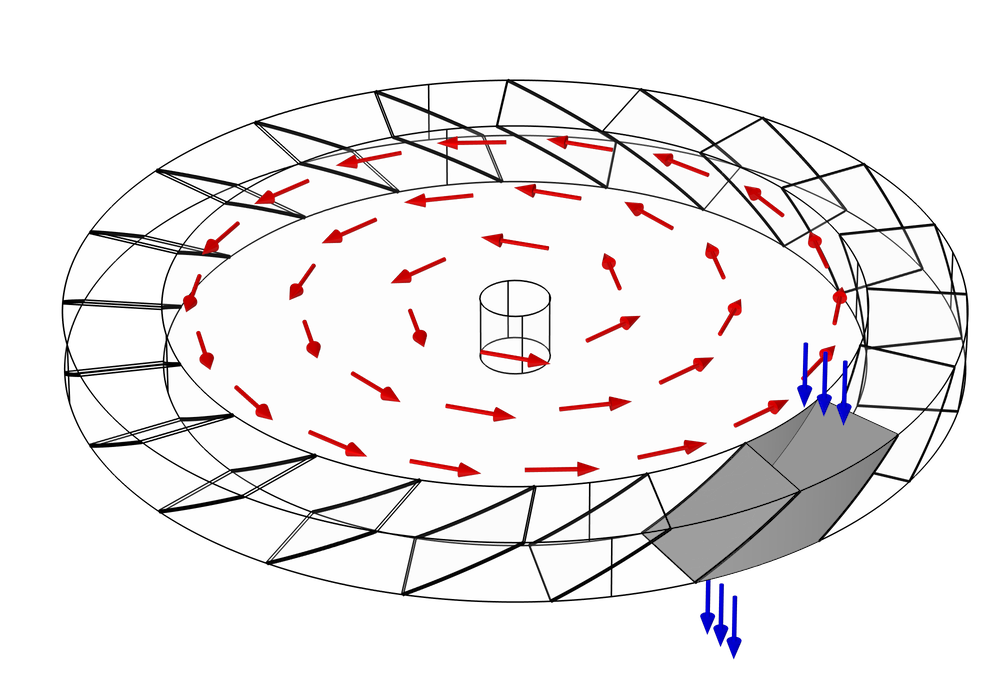### 选择参照系

1. 在“实验室”参考系中建立模型。也就是说，从观察者站在静止不动的外部并观察其旋转的角度对转子进行建模。为此，我们可能必须使用专用的旋转域节点显式地使几何图形随时间旋转。从这个角度来看，分子从一个表面移动到另一个表面时遵循直线路径。
2. 在连接到转子的可移动参考系中建立模型。就是说，想象一下，如果观察者能够缩小并乘坐上这些转子叶片之一，分子的轨迹将如何？在此参考系中，单个气体分子遵循的路径可能看起来是弯曲的。

### 旋转参照系中的粒子追踪

\frac{\textrm{d}}{\textrm{d}t}\left(m_\textrm{p}\frac{\textrm{d}\mathbf{q}}{\textrm{d}t}\right) = \mathbf{F}_\textrm{t}

\frac{R_\textrm{tip}-R_\textrm{root}}{R_\textrm{root}}\ll 1

\begin{align}
\mathbf{F}_\textrm{t} &= \mathbf{F}_\textrm{cen} + \mathbf{F}_\textrm{cor}\\
\mathbf{F}_\textrm{cen} &= m_\textrm{p}\mathbf{\Omega}\times\left(\mathbf{q}\times\mathbf{\Omega}\right)\\
\mathbf{F}_\textrm{cor} &= 2m_\textrm{p}\mathbf{v}\times\Omega
\end{align}

### 粒子释放和边界条件

f(\theta,\phi)\textrm{d}\theta\textrm{d}\phi \propto \sin \theta \cos \theta \textrm{d}\theta\textrm{d}\phi \qquad f(V) = \left(\frac{m_\textrm{p}}{k_\textrm{B}T}\right)^2\frac{V^3}{2}\textrm{exp}\left(-\frac{m_\textrm{p}V^2}{2k_\textrm{B}T}\right)

### 结果

K_\textrm{max} = \frac{M_{12}}{M_{21}} \qquad W_\textrm{max} = M_{12}-M_{21}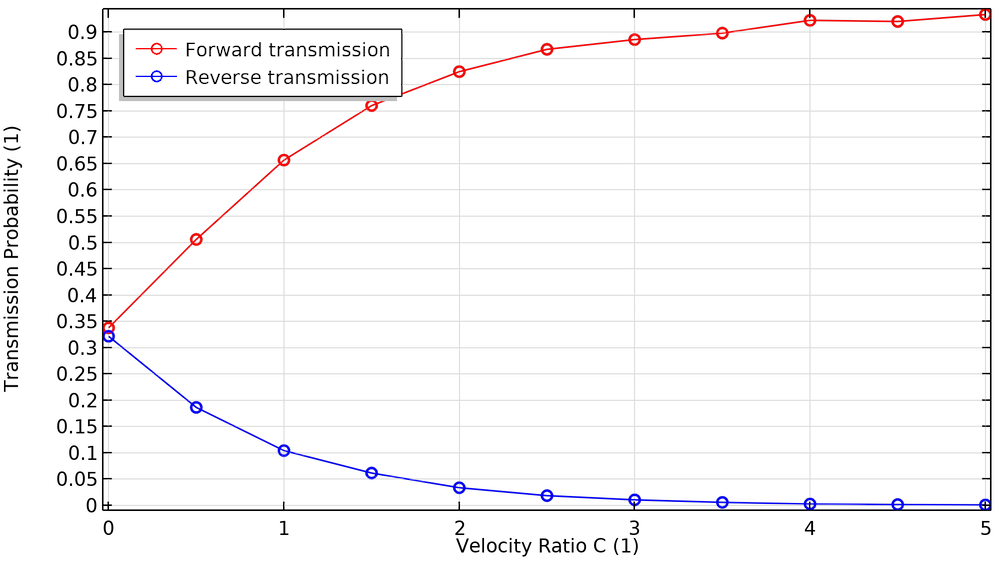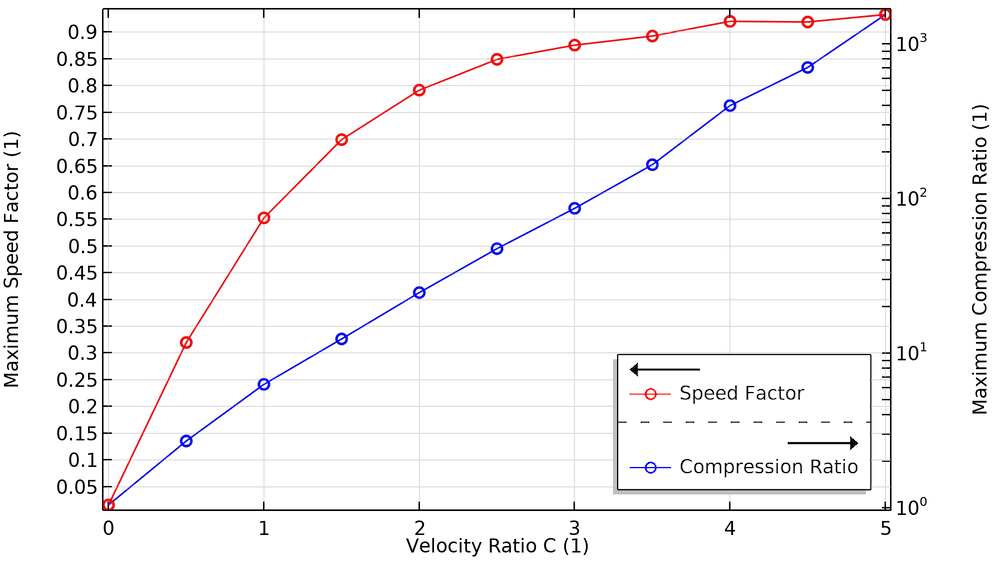#### 了解有关 COMSOL Multiphysics® 中粒子追踪的更多信息

1. J.M. Lafferty, ed., Foundations of Vacuum Science and Technology, John Wiley & Sons, 1998.
2. J.F. O’Hanlon, A User’s Guide to Vacuum Technology, 3rd ed., John Wiley & Sons, 2003.
3. S. Katsimichas, A.J.H. Goddard, R. Lewington, and C.R.E. De Oliveira, “General geometry calculations of one-stage molecular flow transmission probabilities for turbomolecular pumps,” Journal of Vacuum Science & Technology A: Vacuum, Surfaces, and Films, vol. 13, no. 6, pp. 2954–2961, 1995.
4. Y. Li, X. Chen, Y. Jia, M. Liu, and Z. Wang, “Numerical investigation of three turbomolecular pump models in the free molecular flow range,” Vacuum, vol. 101, pp. 337–344, 2014.